# Internal Rate of Return (IRR)

Guide to Understanding the Internal Rate of Return (IRR) Concept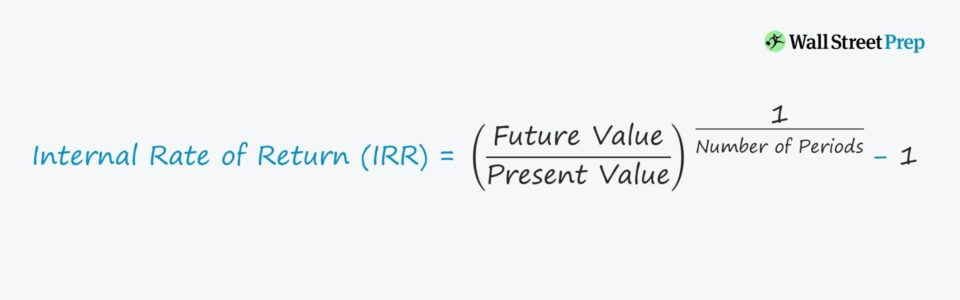• What does the internal rate of return (IRR) measure?
• What formula in Excel calculates the internal rate of return (IRR)?
• Why should both the IRR and MoM be considered when evaluating a potential investment?
• Why is it necessary to take into account both the IRR and MoM?

## How to Calculate the Internal Rate of Return (IRR)

All else being equal, the higher the internal rate of return (IRR), the more benefits (profits) a potential investment will likely bring to a firm if undertaken.

The internal rate of return (IRR) metric estimates the annualized rate of return that an investment is going to yield.

Unlike the MoM, the IRR is considered to be “time-weighted” because it accounts for the specific dates that the cash proceeds are received.

The calculation of the IRR involves the following steps:

1. The future value (FV) is divided by the present value (PV)
2. The amount is raised to the inverse power of the number of periods (i.e., 1 ÷ n)
3. From the resulting figure, one is subtracted

## Shortcomings of Internal Rate of Return (IRR)

The internal rate of return (IRR) is imperfect and cannot be used as a standalone measure due to being very sensitive to timing.

For example, if a private equity firm were to issue itself a dividend soon after a leveraged buyout (LBO), this would increase the IRR to the fund regardless of whether the multiple-of-money (MoM) meets the required returns hurdles – which causes the IRR to be potentially misleading as a result.

However, the IRR could potentially be misleading in its portrayal of returns.

For instance, a high IRR calculated during a short holding period can be artificially inflated and be unsustainable if the holding period were to be extended longer – and thus, IRR cannot be singularly used to make an investment decision.

Let’s say an investment would yield a 30% IRR if sold on the present date, which from first glance sounds great.

But from a more in-depth look, if the same investment achieves only 1.5x MoM, this makes the return far less impressive and the high IRR is attributed more towards a quicker return of capital rather than substantial growth in the size of the investment.

Nonetheless, the IRR tends to be the most benchmarked marketing metric for the performance of investment funds.

For this reason, most firms pay a significant amount of attention to their fund’s IRR.

## Internal Rate of Return (IRR) Levers

The following factors are the main contributors which drive the internal rate of return (IRR):

 Positive IRR Levers Negative IRR Levers Earlier Extraction of Exit Proceeds (e.g., Dividend Recapitalization, Monitoring Fees) Delayed Receipt of Exit Proceeds (e.g., Sale Delay Caused by Lack of Interested Buyers) Increased Free Cash Flows from Strong Revenue and EBITDA Growth Reduced Free Cash Flows and Profit Margins Multiple Expansion (i.e., Exiting at Higher Multiple than Entry) Multiple Contraction (i.e., Lower Exit Multiple than Purchase Multiple)

Regardless, the internal rate of return (IRR) and MoM are both different pieces to the same puzzle, and each comes with its respective shortcomings.

The takeaway is that the magnitude by which an investment grows matters, but the pace at which the growth was achieved is just as important.

## IRR Calculator – Excel Template

We’ll now move to an internal rate of return (IRR) modeling exercise, which you can access by filling out the form below.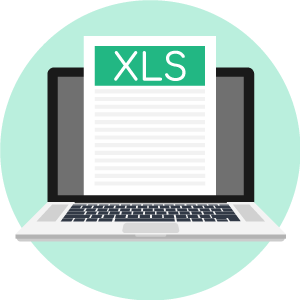Submitting ...

## Internal Rate of Return (IRR) Calculation Example

In our simple example, the equity investment in Year 0 remains fixed at \$85m.

Regardless of which year the firm exits the investment, the value of the initial investment stays unchanged.

Since the investment represents an outflow of cash, we’ll place a negative sign in front of the number.

Meanwhile, the positive cash inflows related to the exit represent the proceeds distributed to the investor following the sale of the investment (i.e., realization at exit).

Here, the assumption is that each year, the exit proceeds will increase by \$25m, starting from the initial investment amount of \$85m. As a result, the exit proceeds in Year 1 are \$110m while in Year 3, the proceeds come out to \$160m.

Once our table depicting the cash outflow in Year 0 (the initial investment) and the cash inflows (the exit proceeds) at different dates in the holding period is done, we can calculate the IRR and MoM metrics from this particular investment.

To determine the internal rate of return (IRR) of an investment, follow the steps below.

1. Start by listing out the value of all of the cash inflows/(outflows) and the corresponding dates of the date of receipt
2. Use the XIRR Excel function (“= XIRR (Range of CFs, Range of Timing)”); the first input requires you to drag the selection box across the range of cash inflows/(outflows)
3. For the second input, do the same across all of the corresponding dates.

Note that the initial cash outflow (initial equity contribution) must be entered as a negative number since the investment is an outflow of cash.

In addition, for the formula to work and be dragged down, the date selection must be anchored in Excel.

###### XIRR vs IRR Excel Function

The XIRR Excel function is preferable over the IRR function as it has more flexibility by not being restricted to annual periods. The drawback to the IRR function is that Excel assumes each cell is separated by precisely twelve months, which is not always the case.

Under XIRR, daily compounding is assumed, and the effective annual rate is returned. But for the IRR function, the interest rate is returned assuming a stream of equally spaced cash flows.

While the two main factors are the entry investment and exit sale proceeds, other inflows such as dividends or monitoring fees (i.e., portfolio company consulting) must be input as positives, as well as any additional equity injections later on in the holding period.

The XIRR function can handle complex scenarios that necessitate taking into account the timing of each cash inflow and outflow (i.e., the volatility of multiple cash flows).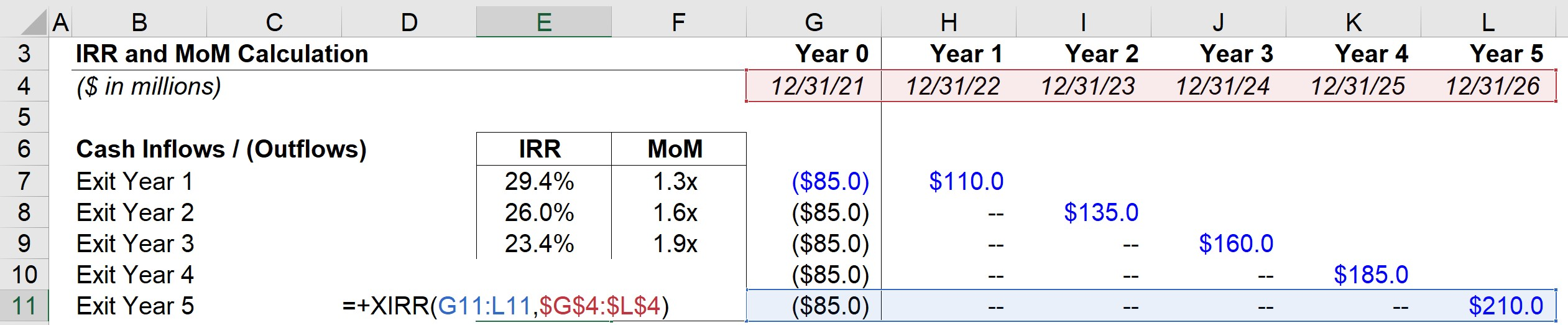To calculate the MoM, we first sum up the cash inflows from the relevant year.

Then, we divide this amount by the cash outflow in Year 0. For instance, assuming a Year 5 exit, the exit proceeds of \$210m are divided by -\$85m to get an MoM of 2.5x.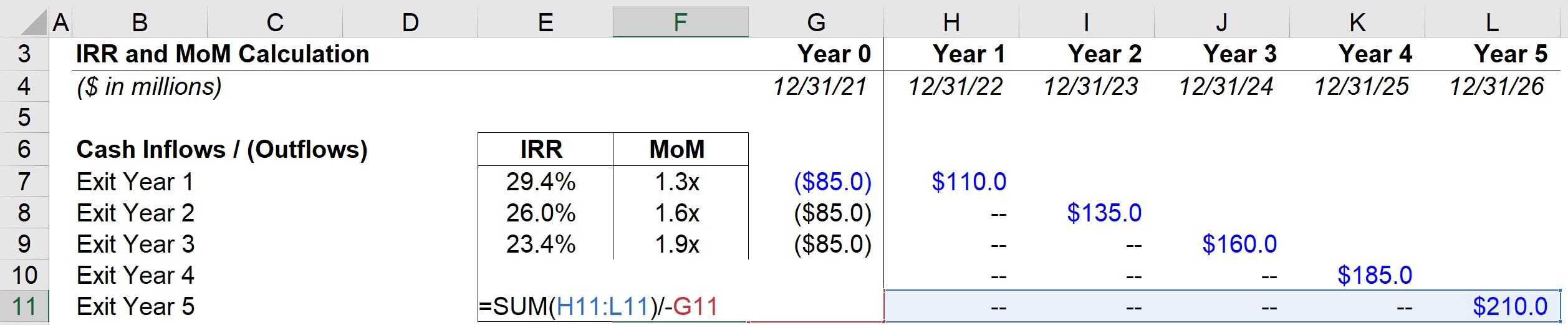Based on the completed output for our exercise, we can see the Year 5 IRR is 19.8% whereas the MoM is 2.5x.

If we were to calculate the IRR using a calculator, the formula would take the future value (\$210m) and divide by the present value (-\$85m) and raise it to the inverse number of periods (1 ÷ 5 Years), and then subtract out one – which again gets us 19.8% for the Year 5 internal rate of return (IRR).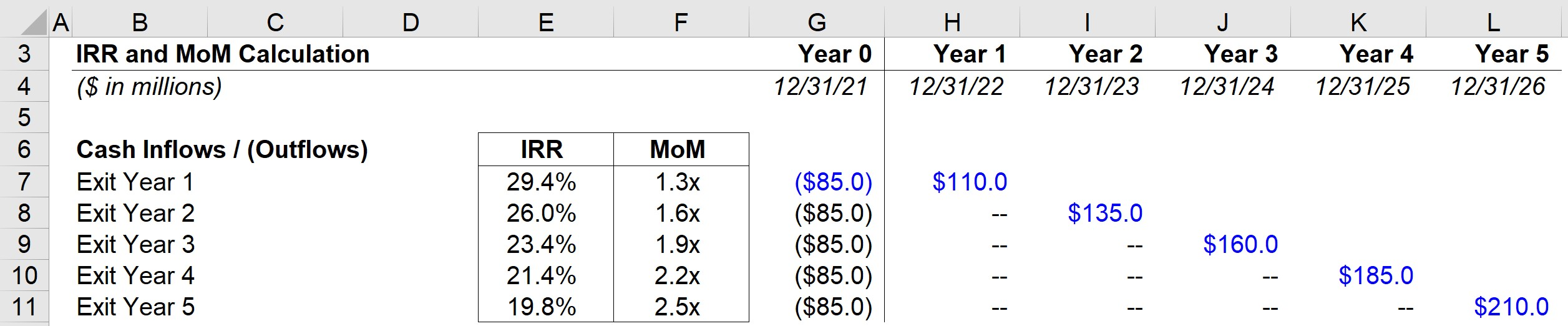Step-by-Step Online Course

#### Everything You Need To Master Financial Modeling

Enroll in The Premium Package: Learn Financial Statement Modeling, DCF, M&A, LBO and Comps. The same training program used at top investment banks.Inline FeedbacksGreg Kleehammer
January 18, 2022 6:34 pm

Re: IRR   For the calculation of IRR denoted on the IRR & MoM Calculation worksheet and explained in the Internal Rate of Return (IRR) document, are the Cash Flows in Years1 through Year 5 Pre-tax or After-tax? Example: Let’s assume that the \$85m is the full cash investment. If the… Read more »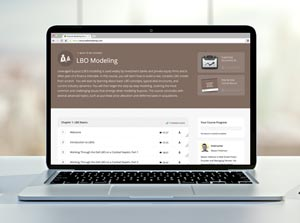Learn LBO Modeling Online

Designed for investment banking and private equity professionals who want to take their LBO modeling skills to the next level.

X

The Wall Street Prep Quicklesson Series

7 Free Financial Modeling Lessons

Get instant access to video lessons taught by experienced investment bankers. Learn financial statement modeling, DCF, M&A, LBO, Comps and Excel shortcuts.